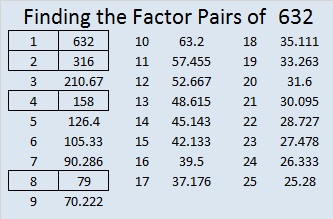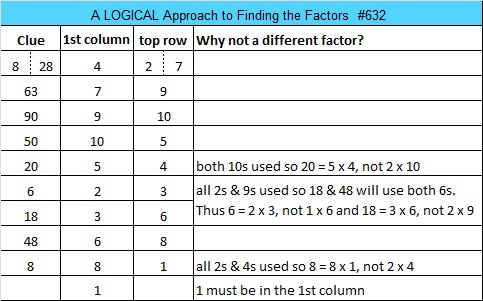# 632 and Level 4

Since 632 is greater than 120 and is divisible by 8 but not by 16, it can be written as the sum of 16 consecutive counting numbers:

32 + 33 + 34 + 35 + 36 + 37 + 38 + 39 + 40 + 41 + 42 + 43 + 44 + 45 + 46 + 47 = 632Print the puzzles or type the solution on this excel file: 10 Factors 2015-09-28

—————————————————————————————————

• 632 is a composite number.
• Prime factorization: 632 = 2 x 2 x 2 x 79, which can be written 632 = (2^3) x 79
• The exponents in the prime factorization are 3 and 1. Adding one to each and multiplying we get (3 + 1)(1 + 1) = 4 x 2 = 8. Therefore 632 has exactly 8 factors.
• Factors of 632: 1, 2, 4, 8, 79, 158, 316, 632
• Factor pairs: 632 = 1 x 632, 2 x 316, 4 x 158, or 8 x 79
• Taking the factor pair with the largest square number factor, we get √632 = (√4)(√158) = 2√158 ≈ 25.13961.—————————————————————————————————This site uses Akismet to reduce spam. Learn how your comment data is processed.